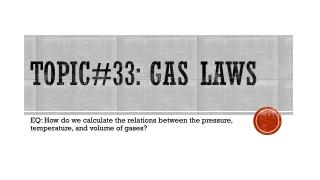DownloadDownload PresentationTopic#33: Gas Laws

# Topic#33: Gas Laws

Télécharger la présentation## Topic#33: Gas Laws

- - - - - - - - - - - - - - - - - - - - - - - - - - - E N D - - - - - - - - - - - - - - - - - - - - - - - - - - -
##### Presentation Transcript

1. Topic#33: Gas Laws EQ: How do we calculate the relations between the pressure, temperature, and volume of gases?

2. Variables: • Volume is measured in Liters, mL, cm3, or dm3 • Temperature can be measured in degrees Farenheit, Celsius or Kelvin, but must be ultimately converted to Kelvin in order to use the gas law formulas. • Amount can be measured in grams, molecules or moles, but must be converted to moles in order to use it in the gas law formulas. • Pressure can be measured in Atmospheres(atm), kilopascals(kPa), millimeters of mercury (mmHg), Torr (torr), or pounds per square inch(psi). You can use any of these units of pressure as long as you are consistent throughout. • Follow your units!!!

3. Pressure Conversion Factors 1 standard atmosphere (atm) = 760 mm Hg (or torr) 1 standard atmosphere (atm) = 14.7 pounds/in2 (psi) 1 standard atmosphere (atm) = 101.3 kPa (SI unit is PASCAL) Therefore: 1 atm =760 mmHg = 760 Torr=101.3 kPa = 14.7 psi

4. Gas Laws • Boyle’s Law P1V1=P2V2 • Charles’s Law • Gay-Lussac’s Law Temperatures must be in KELVIN. K=⁰C+273

5. Example 1 If the temperature of a 12L gas sample increases from 32C to 60C, what will happen to the volume of the gas?

6. Example 2 • If a skin diver takes a breath at the surface, where the pressure is 1 atm, filling his lungs with 5.82 L of air, what volume will the air in his lungs occupy when he dives to a depth where the pressure is 1.92 atm?

7. Example 3 • The pressure of a gas in a tank is 3.20 atm at 22.0⁰C. If the temperature rises to 60.0⁰C, what will be the gas pressure in the tank?

8. Combined Gas Law

9. Example 4 • A gas has a volume of 675 mL at 35°C and 0.850 atm pressure. What is the temperature in °C when the gas has a volume of 0.315 L and a pressure of 802 mm Hg?